## Tamilnadu Samacheer Kalvi 12th Maths Solutions Chapter 5 Two Dimensional Analytical Geometry – II Ex 5.1

12th Maths Exercise 5.1 Samacheer Kalvi Question 1.
Obtain the equation of the circles with radius 5 cm and touching x-axis at the origin in general form.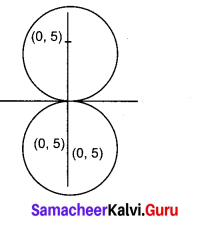Solution:
Given radius = 5 cm and the circle is touching x axis
So centre will be (0, ± 5) and radius = 5
The equation of the circle with centre (0, ± 5) and radius 5 units is
(x – 0)2 + (y ± 5)2 = 52
(i.e) x2 + y2 ± 10 y + 25 – 25 = 0
(i.e) x2 + y2 ± 10y = 0

12th Maths Exercise 5.1 Question 2.
Find the equation of the circle with centre (2, -1) and passing through the point (3, 6) in standard form.
Solution:
Centre = C = (2, -1); Passing through = A = (3, 6)
So radius = CA = $$\sqrt{(2-3)^{2}+(-1-6)^{2}}=\sqrt{1+49}=5 \sqrt{50}$$
Now centre = (2, -1) and radius = $$\sqrt{50}$$
So equation of the circle is
(i.e) (x – 2)2 + (y + 1)2 = $$\sqrt{50}^{2}$$ ⇒ (x – 2)2 + (y + 1)2 = 50

12th Maths Exercise 5.2 Samacheer Kalvi Question 3.
Find the equation of circles that touch both the axes and pass through (-4, – 2) in general form.
Solution:
Since the circle touches both the axes, its centre will be (r, r) and radius wil be r.
Here centre = C = (r, r) and point on the circle is A = (-4, -2)
CA = r ⇒ CA2 = r2
(i.e) (r + 4)2 + (r + 2)2 = r2
⇒ r2 + 8r +16 + r2 + 4r + 4 – r2 = 0
(i.e) r2 + 12r + 20 = 0
(r + 2) (r + 10) = 0
⇒ r = -2 or -10
When r = -2, the equation of the circle will be (x + 2)2 + (y + 2)2 = 22
(i.e) x2 + y2 + 4x + 4y + 4 = 0
When r = -10, the equation of the circle will be (x + 10)2 + (y + 10)2 = 102
(i.e) x2 + y2 + 20x + 20y + 100 = 0

12th Maths Chapter 5 Exercise 5.1 Question 4.
Find the equation of the circle with centre (2, 3) and passing through the intersection of the lines 3x – 2y -1 = 0 and 4x + y – 27 = 0 .
Solution:
To find the point of intersection of the two lines we have to solve the two equations.
Now solving them: 3x — 2y = 1 ……….. (1)
4x + y = 27 ……….(2)
(2) × 2 ⇒ 8x + 2y = 54 ……….. (3)
(1) ⇒ 3x – 2y = 1 ………. (1)
(3) + (1) ⇒ 11x = 55 ⇒ x = $$\frac{55}{11}$$ = 5
Substituting x = 5 in (2) we get
20 + y = 27 ⇒ y = 27 – 20 = 7
∴ The point = A = (5, 7)
Given centre = C = (2, 3)
∴ radius = $$\sqrt{(5-2)^{2}+(7-3)^{2}}=\sqrt{9+16}=5$$ = 5
Now centre = (2, 3) and radius = 5
So equation of the circle is
(x – 2)2 + (y – 3)2 = 52
(i.e) x2 + y2 – 4x – 6y – 12 =0

Class 12 Maths Chapter 5 Exercise 5.1 Question 5.
Obtain the equation of the circle for which (3, 4) and (2, -7) are the ends of a diameter.
Solution:
The equation of a circle with (x1 , y1) and (x2 , y2 ) as end points of a diameter is
(x – x1 )(x – x2) + (y – y1 )(y – y2) = 0
Here the end points of a diameter are (3, 4) and (2, -7)
So equation of the circle is (x – 3 )(x – 2 ) + (y – 4) (y + 7.) = 0
x2 + y2 – 5x + 37 – 22 = 0

Two Dimensional Analytical Geometry 2 Question 6.
Find the equation of the circle through the points (1, 0), (-1, 0), and (0, 1).
Solution:
Let the required circle be
x2 + y2 + 2gx + 2fy + c = 0 …………. (A)
The circle passes through (1, 0), (-1, 0) and (0, 1)
(1, 0) ⇒ 1 + 0 + 2g(1) + 2f(0) + c = 0
2g + c = -1 ……………. (1)
(-1, 0) ⇒ 1 + 0 + 2g (-1) + 2f(0) + c = 0
-2g + c = -1 ……….. (2)
(0, 1) ⇒ 0 + 1 + 2g (0) + 2f(1) + c = 0
2f+ c = -1 ……….. (3)
Now solving (1), (2) and (3) .
2g + c = -1 ………… (1)
-2g + c = -1 ………….. (2)
(1) + (2) ⇒ 2c = -2 ⇒ c = -1
Substituting c = -1 in (1) we get
2g – 1 = -1
2g = -1 + 1 = 0 ⇒ g = 0
Substituting c = -1 in (3) we get
2f – 1 =-1 ⇒ 2f = -1 + 1 = 0 ⇒ f = 0
So we get g = 0, f= 0 and c = -1
So the required circle will be
x2 + y2 + 2(0) x + 2(0)y – 1 = 0
(i.e) x2 + y2 – 1 = 0 ⇒ x2 + y2 = 1

Exercise 5.1 Class 12 Question 7.
A circle of area 9n square units has two of its diameters along the lines x + y = 5 and x – y = 1. Find the equation of the circle.
Solution:
Area of the circle = 9π
(i.e) πr2 = 9π
⇒ r2 = 9 ⇒ r = 3
(i.e) radius of the circle = r = 3
The two diameters are x + y = 5 and x – y = 1
The point of intersection of the diameter is the centre of the circle = C
To find C: Solving x + y = 5 ……… (1)
x – y = 1 ………. (2)
(1) + (2) ⇒ 2x = 6 ⇒ x = 3
Substituting x = 3 in (1) we get
3 + y = 5 ⇒ y = 5 – 3 = 2
∴ Centre = (3, 2) and radius = 3
So equation of the circle is (x – 3)2 + (y – 2)2 = 32
(i.e) x2 + y2 – 6x – 4y + 4 =0

Ex 5.1 Class 12 Question 8.
If y = $$2 \sqrt{2} x$$ + c is a tangent to the circle x2 + y2 =16 , find the value of c .
Solution:
The condition for the line y = mx + c to be a tangent to the circle x2 + y2 = a2 is
c2 = a2(1 + m2)
Here x2 + y2 = 16 ⇒ a2 = 16
y = $$2 \sqrt{2} x$$ + c ⇒ m = $$2 \sqrt{2}$$ and c = c
The condition is c2 = a2 (1 + m2)
(i.e) c2 = 16(1 + 8) = 144
⇒ c = ± 12

Chapter 5 Maths Class 12 Question 9.
Find the equation of the tangent and normal to the circle x2 + y2 – 6x + 6y – 8 = 0 at (2, 2).
Solution:
The equation of the tangent to the circle x2 + y2 + 2 gx + 2fy + c = 0 at (x1, y1) is
xx1 + yy1 + g(x + x1) + f(y + y1) + c = 9
So the equation of the tangent to the circle
x2 + y2 – 6x + 6y – 8 = 0 at (x1, y1) is
xx1 + yy1 – $$\frac{6\left(x+x_{1}\right)}{2}+\frac{6\left(y+y_{1}\right)}{2}$$ – 8 = 0
(i.e) xx1 + yy1 – 3(x + x1) + 3(y + y1) – 8 = 0
Here (x1, y1) = (2, 2)
So equation of the tangent is
x(2) + y(2) – 3(x + 2) + 3(y + 2) – 8 = 0
(.i.e) 2x + 2y – 3x – 6 + 3y + 6 – 8 = 0
(i.e) -x + 5y – 8 = 0 or x – 5y + 8=0
Normal is a line ⊥r to the tangent
So equation of normal circle be of the form 5x + y + k = 0
The normal is drawn at (2, 2)
⇒ 10 + 2 + k = 0 ⇒ k = -12
So equation of normal is 5x + y – 12 = 0

Chapter 5 Class 12 Maths Question 10.
Determine whether the points (-2, 1), (0, 0) and (-4, -3) lie outside, on or inside the circle x2 + y2 – 5x + 2y – 5 = 0 .
Solution:
To find the position of a point with regard to a given circle, substitute the point in the equation of the circle if we get a positive value, the point lies outside the circle.
If we get a -ve value the point lies inside the circle and if we get O then the point lies on the circumference of the circle.
The given circle is x2 + y2 – 5x + 2y – 5 = 0 ……….. (1)
Substituting the point (-2, 1) in (1) we get
4 + 1 – 5(-2) + 2(1) – 5 = 5 + 10 + 2 – 5 = 12
⇒ (- 2, 1) lies outside the circle
Substituting the point (0, 0) in (1) we get
-5 < 0 ⇒ (0, 0) lies inside the circle Substituting the point (-4, -3) in (1) we get 16 + 9 + 20 – 6 – 5 = 34 >0
⇒ (- 4, -3) lies outside the circle

12th Maths 5th Chapter Samacheer Kalvi Question 11.
Find centre and radius of the following circles.
(i) x2 + (y + 2)2 = 0
(ii) x2 + y2 + 6x – 4y + 4 = 0
(iii) x2 + y2 – x + 2y – 3 = 0
(iv) 2x2 + 2y2 – 6x + 4y + 2 = 0
Solution:
(i) x2 + (y + 2)2 = 0
(i.e) x2 + y2 + 4y + 4 = 0
Comparing this equation with the general form x2 + y2 + 2gx + 2fy + c = 0
we get 2g = 0 ⇒ g = 0
2f = 4 ⇒ f= 2 and c = 4
Now centre = (-g, -f) = (0, -2)
Radius = r = $$\sqrt{g^{2}+f^{2}-c}=\sqrt{0+4-4}$$
∴ Centre = (0, -2) and radius = 0

(ii) x2 + y2 + 6x – 4y + 4 = 0
Comparing with the general form we get
2g = 6, 2f = -4
⇒ g = 3, /= -2 and c = 4
Centre = (-g, -f) = (-3, 2)
Radius = $$\sqrt{g^{2}+f^{2}-c}=\sqrt{9+4-4}$$= 3
∴ Centre = (-3, 2) and radius = 3

(iii) x2 + y2 – x + 2y – 3 = 0
Comparing with the general form of the circle we get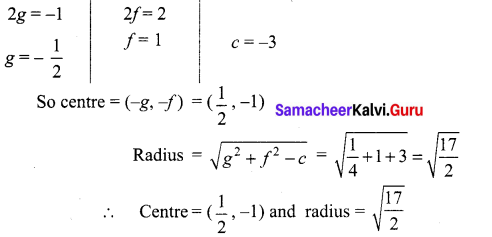(iv) 2x2 + 2y2 – 6x + 4y + 2 = 0
(÷ by 2) ⇒ x2 + y2 – 3x + 2y + 1 =0
Comparing this equation with the general form of the circle we get
2g = -3, 2f= 2
g = $$-\frac{3}{2}$$, g= 1 and c = 1
So centre = (-g, -f) = ($$\frac{3}{2}$$, -1)
and radius = $$\sqrt{g^{2}+f^{2}-c}=\sqrt{\frac{9}{4}+1-1}=\frac{3}{2}$$
∴ Centre = ($$\frac{3}{2}$$, -1) and radius = $$\frac{3}{2}$$

Two Dimensional Analytical Geometry Pdf Question 12.
If the equation 3x2 + (3 -p) xy + qy2 – 2px = 8 pq represents a circle, find p and q. Also determine the centre and radius of the circle.
Solution:
For a circle co-eff of x2 = co-efif of y2
⇒ 3 = q
co-eff of xy = 0
⇒ 3 – p = 0 ⇒ p = 3
So p = q = 3
So the equation of the circle becomes 3x2 + 3y2 – 6x – 72 = 0
(÷ by 3) ⇒ x2 + y2 – 2x – 24 = 0
Comparing this equation with the general form of the circle we get
2g = -2, 2f = 0
g = -1, f = 0 and c = -24
So centre = (-g, -f) = (1, 0) and radius = $$\sqrt{g^{2}+f^{2}-c}$$ = 5
∴ Centre =(1,0) and radius = 5

### Samacheer Kalvi 12th Maths Solutions Chapter 5 Two Dimensional Analytical Geometry – II Ex 5.1 Additional Problems

Ex5 1 Class 12 Question 1.
Find the equation of the circle whose centre is (2, -3) and passing through the intersection of the line 3x – 2y = 1 and 4x + y = 27.
Solution:
Solving 3x – 2y = 1 and 4x+y = 27
Simultaneously, we get x = 5 and y = 7
∴ The point of intersection of the lines is (5, 7)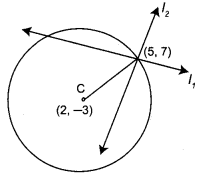Now we have to find the equation of a circle whose centre is (2, -3) and which passes through (5,7)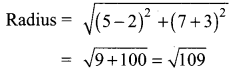∴ Required equation of the circle is
(x – 2)2 + (y + 3)2 = $$(\sqrt{109})^{2}$$
⇒ x2 + y2 – 4x + 6y – 96 = 0

Class 12 Maths Chapter 5 Question 2.
Find the equation of a circle of radius 5 whose centre lies on x-axis and which passes through the point (2, 3).
Solution:
Let the centre of the circle be (h, k). Since the centre lies on x-axis, we have k = 0
Therefore, centre = (h, 0)
Also the circle has radius 5 units and passes through the point (2, 3).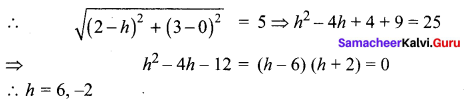Hence the centre is (6, 0) or (-2, 0)
∴ The equation of the circle is
(x – 6)2 + (y – 0)2 = 52 ⇒ x2 + y2 – 12x+ 11 = 0 (Or) (x + 2)2 + (y – 0)2 = 52 ⇒ x2 + y2 + 4x – 21 =0

Two Dimensional Analytical Geometry Question 3.
Find the centre and radius of the following circles:
x2 + y2 – 2x + 4y – 4 = 0 and 2x2 + 2y2 + 16x – 28y + 32 = 0. Also find the ratio of their diameters.
Solution:
Comparing the equation x2 + y2 – 2x + 4y – 4 = 0 with x2 + y2 + 2gx + 2 fy + c = 0, we get
2g = -2 ⇒ g = -1, 2f = 4 ⇒ f = 2, c = -4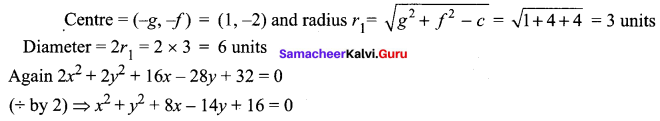Comparing with x2 + y2 + 2gx + 2fy + c = 0
2g = 8 ⇒ g = 4, 2f = -14 ⇒ f = -7, c = 16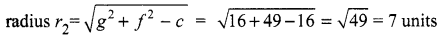Diameter = 2r2 = 2 × 7 = 14 units
Ratio of their diameters = 6 : 14 = 3 : 7.

Class 12 Maths Ex5.1 Question 4.
Find the equation of the circle whose radius is 4 and which is concentric with the circle x2 + y2 + 2x – 6y = 0
Solution:
x2 + y2 + 2x – 6y = 0 …(1)
Here 2g = 2 ⇒ g = 1, 2f = -6 ⇒ f = -3
Centre of the circle = (-g, -f) = (-1, 3)
Since the required circle is concentric with (1), its centre is also (-1, 3).
∴ The equation of the circle whose centre is (-1, 3) and radius 4 is
(x + 1)2 + (y – 3)2 = 42
⇒ x2 + y2 + 2x – 6y – 6 = 0

Exercise 5.1 Class 12 Solutions Question 5.
Show that the four points (1, 0), (2, -7), (8,1) and (9, 6) are concyclic.
Solution:
Let the equation of the circle be Since it passes through (1,0) ⇒ x2 + y2 + 2gx + 2fy + c = 0 …(1)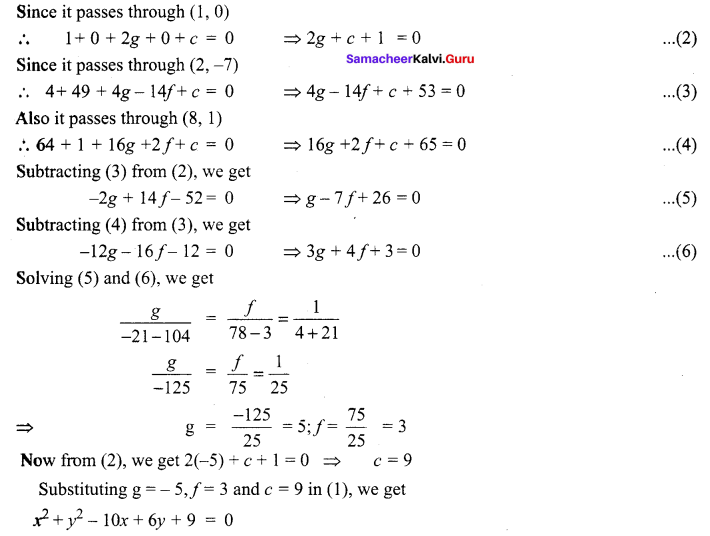Exercise 5.1 Class 12 Maths Question 6.
Find the equation of a circle which passes through the points (1, -2) and (4, -3) and whose centre lies on the line 3x + 4y = 0
Solution:
Let the equation of the circle be
x2 + y2 + 2gx + 2fy + c = 0 …(1)
Since it passes through (1, -2) and (4, -3)
∴ 5 + 2g – 4f + c = 0 …(2)
and 25 + 8g – 6f + c = 0 …(3)
Also (-g, -f) centre of circle (1) lies on 3x + 4y = 7
-3g – 4f = 7 …(4)
Subtracting (2) from (3), we get
20 + 6g – 2f = 0 …(5)
Solving (4) and (5), we get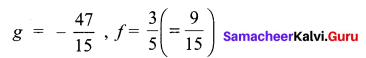Substituting these values of g and f in (2)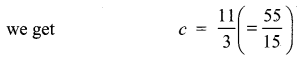∴ From (1), we get,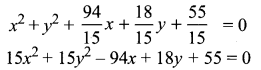Which is the required equation on the circle.

Math Solution Class 12 Chapter 5 Question 7.
Find the equation (s) of the circle passing through the points (1, 1) and (2, 2) and whose radius is 1.
Solution:
Let the equation of the circle be
x2 + y2 + 2gx + 2fy + c = 0 …. (1)
Since it passes through (1,1)
∴ 1 + 1 + 2g + 2f + c = 0
⇒ 2g + 2f + c + 2 = 0 ….(2)
Again it passes through (2, 2)
∴ 4 + 4+ 4g + 4/+ c = 0
⇒ 4g + 4f + c + 8 = 0 …(3)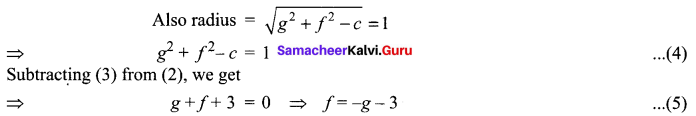g2 + 2g + f2 + 2f + 2 = 1
g2 + 2g + (-g – 3)2 + 2(-g – 3) + 1 = 0
⇒ 2g2 + 6g + 4 = 0 ⇒ g2 + 3g + 2 = 0
⇒ (g + 1)(g + 2) = 0 ⇒ g = -1, -2
when g = -1, from (5), f = – (-1) – 3 = 1 – 3 = -2
when g = -2, from (5), f = – (-2) – 3 = 2 – 3 = -1
Substituting g = -1, f = -2 in (2), we get
2(-1) + 2(-2) + c + 2 =0
⇒ – 6 + c + 2 = 0 ⇒ c = 4
Now putting g = -1, f = -2 and c = 4 in (1), we get
x2 + y2 – 2x – 4y + 4 = 0
Again putting g = -2, f = -1 in (2), we get
2(-2) + 2(-1) + c + 2 =0
⇒ -4 – 2 + c + 2 =0 ⇒ c = 4
Putting g = -2, f = -1 and c = 4 (in) (1), we get
x2 + y2 – 4x – 2y + 4 = 0
Hence the required equation (s) of the circle are
x2 + y2 – 2x – 4y + 4 =0 and x2 + y2 – 4x – 2y + 4 = 0.